Anubhav Sinha

Sector 61 Block E, Noida, India - 201301Featured

# Anubhav Sinha

## Want to Love Maths ? ( for students upto Class XII...

Sector 61 Block E, Noida, India - 201301.

5.0

UrbanPro Rating

Details verified of Anubhav Sinha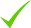IdentityEducation

Know how UrbanPro verifies Tutor details

Identity is verified based on matching the details uploaded by the Tutor with government databases.

## Overview

I have been a sharp student all my life with deep interest in Mathematics and Science. Have secured 99%ile in IITJEE and AIEEE 2011 . In CAT, I achieved 98%ile in 2009.
I completed my engineering in Electronics and Communications and did my MBA from Symbiosis Institute of Business Management, Pune.
After that I have spent close to 8 years in corporate sector in various functions viz. Strategy / Marketing & Operations.
Now, I want to help remove the fear of mathematics from students.
For any teaching requirements for Mathematics in Noida, please reach out to me.

## Languages Spoken

Hindi Mother Tongue (Native)

English Proficient

## Education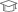Symbiosis Institute of Business Management, Pune 2011

Sector 61 Block E, Noida, India - 201301

## Verified Info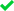ID VerifiedPhone VerifiedEmail VerifiedFacebook Verified

## Demo Class

Yes, not charged

Report this Profile

Is this listing inaccurate or duplicate? Any other problem?

Type the letters as shown below *

Please enter the letters as show below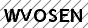## Class 12 Tuition Overview

Class 12 Tuition

Class Location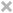Student's HomeTutor's HomeOnline (video chat via skype, google hangout etc)

Years of Experience in Class 12 Tuition

8

Board

State, CBSE, International Baccalaureate, ISC/ICSE, IGCSE

IB Subjects taught

Mathematics

ISC/ICSE Subjects taught

Mathematics

CBSE Subjects taught

Mathematics

IGCSE Subjects taught

Mathematics

Experience in School or College

Teaching is a very satisfying profession through which I help students achieve their goals.

Taught in School or College

Yes

State Syllabus Subjects taught

Mathematics

## Reviews

this is test message this is test message this is test message this is test message this is test message this is test message this is test message

No Reviews yet! Be the first one to Review

## Answers by Anubhav Sinha (1)

If x^2+y^2+z^2=xy+yz+zx then, 2x^2+2y^2+2z^2=2xy+2yz+2zx ie (x^2+y^2) + (y^2+z^2) + (z^2+x^2) = 2xy+2yz+2zx ie (x^2+y^2 -2xy) + (y^2+z^2 -2yz) + (z^2+x^2 - 2zx) = 0 ie (x-y)^2 + (y-z)^2 + (z-x)^2 = 0 , which will happen only when x = y = z = k (let) Now, consider (x+y+z)^3 = x^3 + (y+z)^3 + 3x(y+z) (x+... ...more

If x^2+y^2+z^2=xy+yz+zx then,

2x^2+2y^2+2z^2=2xy+2yz+2zx ie

(x^2+y^2) + (y^2+z^2) + (z^2+x^2) = 2xy+2yz+2zx ie

(x^2+y^2 -2xy) + (y^2+z^2 -2yz) + (z^2+x^2 - 2zx) = 0 ie

(x-y)^2 + (y-z)^2 + (z-x)^2 = 0 ,

which will happen only when

x = y = z = k (let)

Now, consider

(x+y+z)^3 = x^3 + (y+z)^3 + 3x(y+z) (x+ y +z )

=x^3 + (y^3 + z^3 + 3y^2z + 3yz^2 ) +3x (xy + y^2 + yz + xz + yz + z^2)

= x^3 + y^3 + z^3 + 3y^2z + 3yz^2  +3x^2y + 3xy^2 + 3xyz + 3x^2z + 3xyz +3xz^2

= x^3 + y^3 + z^3 + 3y^2z + 3yz^2  +3x^2y + 3xy^2 + 3xyz + 3x^2z + 3xyz +3xz^2

so,

x^3 + y^3 + z^3 = (x+y+z)^3 - (3y^2z +3yz^2  +3x^2y +3xy^2 +3xyz +3x^2z +3xyz +3xz^2)

= (3k)^3 - k^3 (3+3+3+3+3+3+3+3)

= k^3 (27-24)

= 3k^3 = 3x^3 = 3y^3 = 3z^3 = 3x^2y= 3x^2z = 3y^2x = 3y^2z = 3z^2x = 3z^2y = 3xyz

Dislike Bookmark

x
Class 12 Tuition 5.0

Class LocationStudent's HomeTutor's HomeOnline (video chat via skype, google hangout etc)

Years of Experience in Class 12 Tuition

8

Board

State, CBSE, International Baccalaureate, ISC/ICSE, IGCSE

IB Subjects taught

Mathematics

ISC/ICSE Subjects taught

Mathematics

CBSE Subjects taught

Mathematics

IGCSE Subjects taught

Mathematics

Experience in School or College

Teaching is a very satisfying profession through which I help students achieve their goals.

Taught in School or College

Yes

State Syllabus Subjects taught

Mathematics

Class 11 Tuition 5.0

Class LocationStudent's HomeTutor's HomeOnline (video chat via skype, google hangout etc)

Years of Experience in Class 11 Tuition

8

Board

State, CBSE, International Baccalaureate, ISC/ICSE, IGCSE

IB Subjects taught

Mathematics

ISC/ICSE Subjects taught

Mathematics

CBSE Subjects taught

Mathematics

IGCSE Subjects taught

Mathematics

Taught in School or College

No

State Syllabus Subjects taught

Mathematics

Class 10 Tuition 5.0

Class LocationStudent's HomeTutor's HomeOnline (video chat via skype, google hangout etc)

Years of Experience in Class 10 Tuition

8

Board

ICSE, International Baccalaureate, State, CBSE, IGCSE

IB Subjects taught

Mathematics

CBSE Subjects taught

Mathematics

ICSE Subjects taught

Mathematics

IGCSE Subjects taught

Mathematics

Taught in School or College

No

State Syllabus Subjects taught

Mathematics

Class 9 Tuition 5.0

Class LocationStudent's HomeTutor's HomeOnline (video chat via skype, google hangout etc)

Years of Experience in Class 9 Tuition

8

Board

ICSE, International Baccalaureate, State, CBSE, IGCSE

IB Subjects taught

Mathematics

CBSE Subjects taught

Mathematics

ICSE Subjects taught

Mathematics

IGCSE Subjects taught

Mathematics

Taught in School or College

No

State Syllabus Subjects taught

Mathematics

Class 8 Tuition 5.0

Class LocationStudent's HomeTutor's HomeOnline (video chat via skype, google hangout etc)

Years of Experience in Class 8 Tuition

8

Board

CBSE, IGCSE, International Baccalaureate, ICSE, State

IB Subjects taught

Mathematics

CBSE Subjects taught

Mathematics

ICSE Subjects taught

Mathematics

IGCSE Subjects taught

Mathematics

Taught in School or College

No

State Syllabus Subjects taught

Mathematics

this is test message this is test message this is test message this is test message this is test message this is test message this is test message

No Reviews yet! Be the first one to Review

## Answers by Anubhav Sinha (1)

If x^2+y^2+z^2=xy+yz+zx then, 2x^2+2y^2+2z^2=2xy+2yz+2zx ie (x^2+y^2) + (y^2+z^2) + (z^2+x^2) = 2xy+2yz+2zx ie (x^2+y^2 -2xy) + (y^2+z^2 -2yz) + (z^2+x^2 - 2zx) = 0 ie (x-y)^2 + (y-z)^2 + (z-x)^2 = 0 , which will happen only when x = y = z = k (let) Now, consider (x+y+z)^3 = x^3 + (y+z)^3 + 3x(y+z) (x+... ...more

If x^2+y^2+z^2=xy+yz+zx then,

2x^2+2y^2+2z^2=2xy+2yz+2zx ie

(x^2+y^2) + (y^2+z^2) + (z^2+x^2) = 2xy+2yz+2zx ie

(x^2+y^2 -2xy) + (y^2+z^2 -2yz) + (z^2+x^2 - 2zx) = 0 ie

(x-y)^2 + (y-z)^2 + (z-x)^2 = 0 ,

which will happen only when

x = y = z = k (let)

Now, consider

(x+y+z)^3 = x^3 + (y+z)^3 + 3x(y+z) (x+ y +z )

=x^3 + (y^3 + z^3 + 3y^2z + 3yz^2 ) +3x (xy + y^2 + yz + xz + yz + z^2)

= x^3 + y^3 + z^3 + 3y^2z + 3yz^2  +3x^2y + 3xy^2 + 3xyz + 3x^2z + 3xyz +3xz^2

= x^3 + y^3 + z^3 + 3y^2z + 3yz^2  +3x^2y + 3xy^2 + 3xyz + 3x^2z + 3xyz +3xz^2

so,

x^3 + y^3 + z^3 = (x+y+z)^3 - (3y^2z +3yz^2  +3x^2y +3xy^2 +3xyz +3x^2z +3xyz +3xz^2)

= (3k)^3 - k^3 (3+3+3+3+3+3+3+3)

= k^3 (27-24)

= 3k^3 = 3x^3 = 3y^3 = 3z^3 = 3x^2y= 3x^2z = 3y^2x = 3y^2z = 3z^2x = 3z^2y = 3xyz

Dislike Bookmark

Anubhav Sinha describes himself as Want to Love Maths ? ( for students upto Class XII ). He conducts classes in Class 10 Tuition, Class 11 Tuition and Class 12 Tuition. Anubhav is located in Sector 61 Block E, Noida. Anubhav takes Regular Classes- at his Home. He has 8 years of teaching experience . Anubhav has completed Master of Business Administration (M.B.A.) from Symbiosis Institute of Business Management, Pune in 2011. He is well versed in English and Hindi.

Recommended Profiles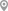Sector 55, NoidaSector 31, NoidaSector-41 Noida, NoidaSector 63, NoidaSector 50 D Block, NoidaSector 53, Noida

X

Let us shortlist and give the best tutors and institutes.

or

Send Enquiry to Anubhav Sinha

Let Anubhav Sinha know you are interested in their class